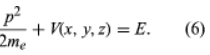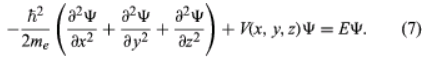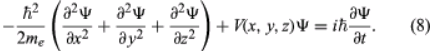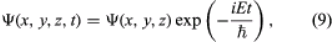Schrodinger's Wave Mechanics

# Schrodinger's Wave Mechanics - Notes | Study Modern Physics for IIT JAM - Physics

 1 Crore+ students have signed up on EduRev. Have you?

Schrodinger's Wave Equation:

Schrodinger expressed de Broglie’s hypothesis concerning the wave behaviour of matter in a mathematical form that is adaptable to a variety of physical problems without additional arbitrary assumptions. He was guided by a mathematical formulation of optics, in which the straight-line propagation of light rays can be derived from wave motion when the wavelength is small compared to the dimensions of the apparatus employed. In the same way, Schrodinger set out to find a wave equation for matter that would give particle-like propagation when the wavelength becomes comparatively small. According to classical mechanics, if a particle of mass me is subjected to a force such that its potential energy is V(x, y, z) at position x, y, z, then the sum of V(x, y, z) and the kinetic energy p2/2me is equal to a constant, the total energy E of the particle. Thus,It is assumed that the particle is bound—i.e., confined by the potential to a certain region in space because its energy E is insufficient for it to escape. Since the potential varies with position, two other quantities do also: the momentum and, hence, by extension from the de Broglie relation, the wavelength of the wave. Postulating a wave function Ψ(x, y, z) that varies with position, Schrödinger replaced p in the above energy equation with a differential operator that embodied the de Broglie relation. He then showed that Ψ satisfies the partial differential equationThis is the (time-independent) Schrödinger wave equation, which established quantum mechanics in a widely applicable form. An important advantage of Schrödinger’s theory is that no further arbitrary quantum conditions need be postulated. The required quantum results follow from certain reasonable restrictions placed on the wave function—for example, that it should not become infinitely large at large distances from the centre of the potential.
Schrödinger applied his equation to the hydrogen atom, for which the potential function, given by classical electrostatics, is proportional to −e2/r, where −e is the charge on the electron. The nucleus (a proton of charge e) is situated at the origin, and r is the distance from the origin to the position of the electron. Schrödinger solved the equation for this particular potential with straightforward, though not elementary, mathematics. Only certain discrete values of E lead to acceptable functions Ψ. These functions are characterized by a trio of integers n, l, m, termed quantum numbers. The values of E depend only on the integers n (1, 2, 3, etc.) and are identical with those given by the Bohr theory. The quantum numbers l and m are related to the angular momentum of the electron; Square root of√l(l + 1)ℏ is the magnitude of the angular momentum, and mℏ is its component along some physical direction.
The square of the wave function, Ψ2, has a physical interpretation. Schrödinger originally supposed that the electron was spread out in space and that its density at point x, y, z was given by the value of Ψ2 at that point. Almost immediately Born proposed what is now the accepted interpretation—namely, that Ψ2 gives the probability of finding the electron at x, y, z. The distinction between the two interpretations is important. If Ψ2 is small at a particular position, the original interpretation implies that a small fraction of an electron will always be detected there. In Born’s interpretation, nothing will be detected there most of the time, but, when something is observed, it will be a whole electron. Thus, the concept of the electron as a point particle moving in a well-defined path around the nucleus is replaced in wave mechanics by clouds that describe the probable locations of electrons in different states.

Electron spin and anti-particles:

In 1928 the English physicist Paul A.M. Dirac produced a wave equation for the electron that combined relativity with quantum mechanics. Schrödinger’s wave equation does not satisfy the requirements of the special theory of relativity because it is based on a nonrelativistic expression for the kinetic energy (p2/2me). Dirac showed that an electron has an additional quantum number ms. Unlike the first three quantum numbers, ms is not a whole integer and can have only the values +1/2 and −1/2. It corresponds to an additional form of angular momentum ascribed to a spinning motion. (The angular momentum mentioned above is due to the orbital motion of the electron, not its spin.) The concept of spin angular momentum was introduced in 1925 by Samuel A. Goudsmit and George E. Uhlenbeck, two graduate students at the University of Leiden, Neth., to explain the magnetic moment measurements made by Otto Stern and Walther Gerlach of Germany several years earlier. The magnetic moment of a particle is closely related to its angular momentum; if the angular momentum is zero, so is the magnetic moment. Yet Stern and Gerlach had observed a magnetic moment for electrons in silver atoms, which were known to have zero orbital angular momentum. Goudsmit and Uhlenbeck proposed that the observed magnetic moment was attributable to spin angular momentum.
The electron-spin hypothesis not only provided an explanation for the observed magnetic moment but also accounted for many other effects in atomic spectroscopy, including changes in spectral lines in the presence of a magnetic field (Zeeman effect), doublet lines in alkali spectra, and fine structure (close doublets and triplets) in the hydrogen spectrum.
The Dirac equation also predicted additional states of the electron that had not yet been observed. Experimental confirmation was provided in 1932 by the discovery of the positron by the American physicist Carl David Anderson. Every particle described by the Dirac equation has to have a corresponding antiparticle, which differs only in charge. The positron is just such an antiparticle of the negatively charged electron, having the same mass as the latter but a positive charge.

Identical particles and multi-electron atoms:

Because electrons are identical to (i.e., indistinguishable from) each other, the wave function of an atom with more than one electron must satisfy special conditions. The problem of identical particles does not arise in classical physics, where the objects are large-scale and can always be distinguished, at least in principle. There is no way, however, to differentiate two electrons in the same atom, and the form of the wave function must reflect this fact. The overall wave function Ψ of a system of identical particles depends on the coordinates of all the particles. If the coordinates of two of the particles are interchanged, the wave function must remain unaltered or, at most, undergo a change of sign; the change of sign is permitted because it is Ψ2 that occurs in the physical interpretation of the wave function. If the sign of Ψ remains unchanged, the wave function is said to be symmetric with respect to interchange; if the sign changes, the function is antisymmetric.
The symmetry of the wave function for identical particles is closely related to the spin of the particles. In quantum field theory (see below Quantum electrodynamics), it can be shown that particles with half-integral spin (1/2, 3/2, etc.) have antisymmetric wave functions. They are called fermions after the Italian-born physicist Enrico Fermi. Examples of fermions are electrons, protons, and neutrons, all of which have spin 1/2. Particles with zero or integral spin (e.g., mesons, photons) have symmetric wave functions and are called bosons after the Indian mathematician and physicist Satyendra Nath Bose, who first applied the ideas of symmetry to photons in 1924–25.
The requirement of antisymmetric wave functions for fermions leads to a fundamental result, known as the exclusion principle, first proposed in 1925 by the Austrian physicist Wolfgang Pauli. The exclusion principle states that two fermions in the same system cannot be in the same quantum state. If they were, interchanging the two sets of coordinates would not change the wave function at all, which contradicts the result that the wave function must change sign. Thus, two electrons in the same atom cannot have an identical set of values for the four quantum numbers n, l, m, ms. The exclusion principle forms the basis of many properties of matter, including the periodic classification of the elements, the nature of chemical bonds, and the behaviour of electrons in solids; the last determines in turn whether a solid is a metal, an insulator, or a semiconductor (see atom; matter).
The Schrödinger equation cannot be solved precisely for atoms with more than one electron. The principles of the calculation are well understood, but the problems are complicated by the number of particles and the variety of forces involved. The forces include the electrostatic forces between the nucleus and the electrons and between the electrons themselves, as well as weaker magnetic forces arising from the spin and orbital motions of the electrons. Despite these difficulties, approximation methods introduced by the English physicist Douglas R. Hartree, the Russian physicist Vladimir Fock, and others in the 1920s and 1930s have achieved considerable success. Such schemes start by assuming that each electron moves independently in an average electric field because of the nucleus and the other electrons; i.e., correlations between the positions of the electrons are ignored. Each electron has its own wave function, called an orbital. The overall wave function for all the electrons in the atom satisfies the exclusion principle. Corrections to the calculated energies are then made, which depend on the strengths of the electron-electron correlations and the magnetic forces.

Time-dependent Schrödinger equation:

At the same time that Schrödinger proposed his time-independent equation to describe the stationary states, he also proposed a time-dependent equation to describe how a system changes from one state to another. By replacing the energy E in Schrödinger’s equation with a time-derivative operator, he generalized his wave equation to determine the time variation of the wave function as well as its spatial variation. The time-dependent Schrödinger equation readsThe quantity i is the square root of −1. The function Ψ varies with time t as well as with position x, y, z. For a system with constant energy, E, Ψ has the formwhere exp stands for the exponential function, and the time-dependent Schrödinger equation reduces to the time-independent form.
The probability of a transition between one atomic stationary state and some other state can be calculated with the aid of the time-dependent Schrödinger equation. For example, an atom may change spontaneously from one state to another state with less energy, emitting the difference in energy as a photon with a frequency given by the Bohr relation. If electromagnetic radiation is applied to a set of atoms and if the frequency of the radiation matches the energy difference between two stationary states, transitions can be stimulated. In a stimulated transition, the energy of the atom may increase—i.e., the atom may absorb a photon from the radiation—or the energy of the atom may decrease, with the emission of a photon, which adds to the energy of the radiation. Such stimulated emission processes form the basic mechanism for the operation of lasers. The probability of a transition from one state to another depends on the values of the l, m, ms quantum numbers of the initial and final states. For most values, the transition probability is effectively zero. However, for certain changes in the quantum numbers, summarized as selection rules, there is a finite probability. For example, according to one important selection rule, the l value changes by unity because photons have a spin of 1. The selection rules for radiation relate to the angular momentum properties of the stationary states. The absorbed or emitted photon has its own angular momentum, and the selection rules reflect the conservation of angular momentum between the atoms and the radiation.

The document Schrodinger's Wave Mechanics - Notes | Study Modern Physics for IIT JAM - Physics is a part of the Physics Course Modern Physics for IIT JAM.
All you need of Physics at this link: Physics

## Modern Physics for IIT JAM

54 videos|44 docs|15 tests
 Use Code STAYHOME200 and get INR 200 additional OFF

## Modern Physics for IIT JAM

54 videos|44 docs|15 tests

Track your progress, build streaks, highlight & save important lessons and more!

,

,

,

,

,

,

,

,

,

,

,

,

,

,

,

,

,

,

,

,

,

;